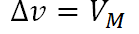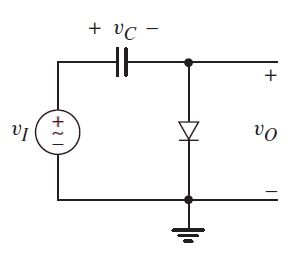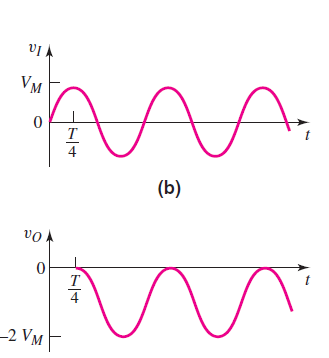# Clamper circuit using a capacitor Calculator

This CalcTown calculator calculates the shift in the output signal using a clamping circuit.Here a negative value of  ?V means a negative shift in the voltage levels.

*Please see the output voltage waveform below.

V

#### Result

V